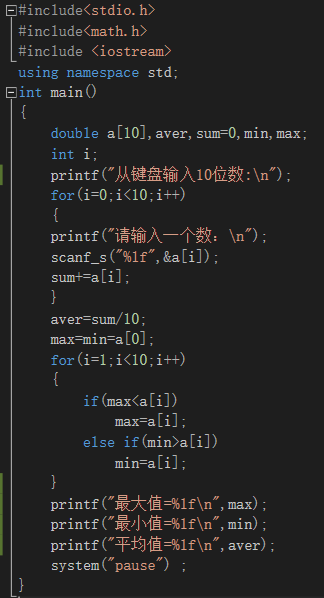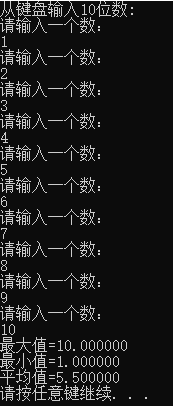• 十个数求最大值
千次阅读
2021-05-18 09:55:10

满意答案

可以用一个循环解决问题，这样代码量少一些，速度也快一些

另外上面有人提供的代码把平均值设置为整数了，平均数应该用float这样才可以包含小数。

#include

#include

void main()

{

int n={1,2,3,4,5,6,7,8,9,10}; //我这里就直接给出数字了，你当然也可以改用任何方法输入或者从文件读入数据

int min, max, n_min, n_max, i;

float avg;

min=n;

max=n;

n_min=0;

n_max=0;

avg=n;

for (i=1; i<10; i++)

{

if (max

{

max=n[i];

n_max=i;

}

else if (min>n[i])

{

min=n[i];

n_min=i;

}

avg=avg+n[i]; ///最后再来除以10

}

avg=avg/10.0;

printf("最大值是n[%d]=%d\n最小值是n[%d]=%d\n平均值=%f\n", n_max, max, n_min, min, avg);

}

10分享举报

更多相关内容
• JAVA编写程序,从10个数最大值,最小值及平均值(急！)43 解决方案#1这问题有点太.....楼主是做XXX的吗？如果是转行的还可以理解。。。#2作业题.....#3你说你学过程序设计没，排序写过吧，你排序后不就得到最大...

JAVA 编写程序,从10个数中求出最大值,最小值及平均值(急！)

43 个解决方案

#1

这问题有点太..... 楼主是做XXX的吗？ 如果是转行的还可以理解。。。

#2

作业题.....

#3

你说你学过程序设计没，排序写过吧，你排序后不就得到最大值和最小值了么，再求和除以10不就ok了

#4

呵呵,弄几个方法,来个赋值循环,最后再求个平均值OK,LZ好好想想

#5

确实很基础啊，LZ要努力啊，

int[] arr={2,34,343,54,67,78,34,12,56,6};

int max=arr;

int min=arr;

int sum=0;

for(int i:arr){

if(i>max){

max=i;

}

if(i

min=i;

}

sum+=i;

}

System.out.println("max:"+max+";\nmin:"+min+"\naverage:"+(sum/arr.length));

#6

引用 1 楼 java2000_net 的回复:

这问题有点太..... 楼主是做XXX的吗？ 如果是转行的还可以理解。。。

刚上路...鼓励一下LZ

#7

public class Ti   //这是个十个数值求最大最小和和的程序

{

public static void main(String[] args)

{

int[] arr={2,42,21,65,3,15,30,58,47,68};

int max=arr;

int min=arr;

int sum=0;

int i=0;

for(i=0;i

{

if (max

{

max=arr[i];

}

if(min>arr[i])

{

min=arr[i];

}

sum=sum+arr[i];

}

System.out.println("max:"+max);

System.out.println("min: "+min);

System.out.println("sum: "+sum);

}

}

#8

mark

#9

来看看，不说话。。。-_-

#10

用sort(),然后取出两个边界值就是最大最小值了~

#11

用Arrays.sort(int[] a)   第一个是最小值，最后一个是最大值，10个数相加除以10得出平均值！

鼓励一下楼主，都是从不会开始的！

#12

用Arrays.sort(int[] a)   第一个是最小值，最后一个是最大值，10个数相加除以10得出平均值！

鼓励一下楼主，都是从不会开始的！

这个方法好,值得学习

#13

楼下继续

#14

哈哈，当年我也是这样的。慢慢来

#15

如果说刚入门，排序不会可以理解，可是这个平均值不会就有点...

#16

这个不是计算机问题,是数学问题...

难怪学数学的鄙视学计算机的呢....哎

#17

不会很正常啊，人都是从不会到会的，可能是没有思路，多想想再加点提示，应该可以实现的。和LZ一起学习~

#18

引用 15 楼 ZX_ARES 的回复:

如果说刚入门，排序不会可以理解，可是这个平均值不会就有点...

同意哈…………建议楼主多看看书，上课认真听讲！！！

#19

其实不教你这种问题才是帮助你！

#20

public class sort implements Comparator

{public int compare(Object o1, Object o2) {

int j1=((Integer)o1).intValue();

int j2=((Integer)o2).intValue();

return j1>j2?1:(j1==j2?0:-1);

}

public static void main(String[] args)

{Integer arr[] = {2,34,343,54,67,78,34,12,56,6};

Arrays.sort(arr,new sort());

System.out.println("Min" + arr);

System.out.println("Max" + arr);

}

}

#21

引用 7 楼 ziwopengzhang 的回复:

public class Ti   //这是个十个数值求最大最小和和的程序

{

public static void main(String[] args)

{

int[] arr=new int

for(i=0;i

array[i]=(int)(Math.random()*100);

int max=arr;

int min=arr;

int sum=0;

int i=0;

for(i=0;i

{

if (max

{

max=arr[i];

}

if(min>arr[i])

{

min=arr[i];

}

sum=sum+arr[i];

}

System.out.println("max:…

你好，请问我这么改可以吗？

#22

class MaxMin{

public static void main(String args[]){

int arr[]={7,2,3,4,6,1,5,8,9,10};

int max=arr;

int min=arr;

int sum=0;

int average=0;

for(int i=0; i

if(max

max=arr[i];

}

if(min>arr[i]){

min=arr[i];

}

sum=sum+arr[i];

average=sum/arr.length;

}

System.out.println(max+" "+min+" "+average);

}

}

#23

引用 11 楼 ThirdDimension 的回复:

用Arrays.sort(int[] a)   第一个是最小值，最后一个是最大值，10个数相加除以10得出平均值！

鼓励一下楼主，都是从不会开始的！

这个不好，效率较低，求极值没有必要排序

#24

引用 7 楼 ziwopengzhang 的回复:

public class Ti   //这是个十个数值求最大最小和和的程序

{

public static void main(String[] args)

{

int[] arr={2,42,21,65,3,15,30,58,47,68};

int max=arr;

int min=arr;

int sum=0;

int i=0;

for(i=0;i

{

if (max

{

max=arr[i];

}

if(min>arr[i])

{

min=arr[i];

}

sum=sum+arr[i];

}

System.out.println("max:…

#25

你需要的是一个编程思想.

排序时,你先定义出3个变量,分别是min,max,sum,对应是用来记录表示最小值,最大值,总和(求平均值时用)

好.

1.先取出第一个数one,用min=one,max=one,sum=one,也就是说如果数组里面仅有一个值时,那么不论最大,最小,平均,总和都是它.对吧?

2.找到下一个数two,对min来说,如果twomax,那么max=two,max始终记录最大的值.sum = sum+two,那么sum的值就是one+two,记录总和.

3.循环第2个过程,不断取下一个值,直到数组取完了.

最后min,max,sum/数组个数 就是你想要的最小,最大,平均值了.

#26

class Test{

static double getMax(double[] array)

{

double max = array;

for(int i = 1;i

if(max

{

max = array[i];

}

}

return max;

}

static double getMin(double[] array)

{

double min = array;

for(int i = 1;i

if(min > array[i])

{

min = array[i];

}

}

return min;

}

static double getAverage(double[] array)

{

double sum = 0;

for(int i = 0;i

sum += array[i];

}

return sum/array.length;

}

public static void main(String[] args) {

double[] array = {9,1,1,2,3,2,2,56,7,4,3};

System.out.println(getMax(array));

System.out.println(getMin(array));

System.out.println(getAverage(array));

}

}

#27

import java.util.Arrays;

public class Test{

static double getMax(double[] array)

{

Arrays.sort(array);

return array[array.length-1];

}

static double getMin(double[] array)

{

Arrays.sort(array);

return array;

}

static double getAverage(double[] array)

{

double sum = 0;

for(double temp:array)

sum += temp;

return sum/array.length;

}

public static void main(String[] args) {

double[] array = {56,2,75,3,7,3,6,8,4,34};

System.out.println(getMax(array));

System.out.println(getMin(array));

System.out.println(getAverage(array));

}

}

#28

int [] ary = {1,34,2,5,2,1,3,65,2,3,4,2,1,1,2,3,4};

int min = ary;

int max = ary;

int sum = 0;

for (int i:ary) {

max=(i>max)?i:max;

min=(i

sum += i;

}

System.out.println(max);

System.out.println(min);

System.out.println(sum/ary.length);

#29

引用 3 楼 zuoguodang 的回复:

你说你学过程序设计没，排序写过吧，你排序后不就得到最大值和最小值了么，再求和除以10不就ok了

#30

用arrays.sort()应该比较方便

#31

看你这么老了，也不要这么小看我们这些刚出来的，你当年也还不是这样！

引用 1 楼 java2000_net 的回复:

这问题有点太..... 楼主是做XXX的吗？ 如果是转行的还可以理解。。。

#32

import java.util.*;

public class gg {

/**

* @param args

*/

public static void main(String[] args) {

// TODO Auto-generated method stub

int[] arr={2,42,21,65,3,15,30,58,47,68};

Arrays.sort(arr);

System.out.println(arr);//最小值

System.out.println(arr[arr.length-1]);//最大值

System.out.println(average(arr));平均值

}

public static double average(int[] a)

{   double sum=0.0;

for(int i=0;i

sum=sum+a[i];

return sum/a.length ;

}

#33

路过。。。

#34

呵呵，真谢谢大家的关注，自己没学过一点JAVA，什么也不懂，只为了帮朋友找作业答案。

#35

求平均值的时候都是：sum/length，如果定义的sum是int型，得到的值只是整数部分，而并不是平均值。

#36

int [] ary = {1,34,2,5,2,1,3,65,2,3,4,2,1,1,2,3,4};

int min = ary;

int max = ary;

int sum = 0;

for (int i:ary) {

max=(i>max)?i:max;

min=(i

sum += i;

}

System.out.println(max);

System.out.println(min);

System.out.println(sum/ary.length);

这个简单

#37

引用 25 楼 myminer 的回复:

你需要的是一个编程思想.

排序时,你先定义出3个变量,分别是min,max,sum,对应是用来记录表示最小值,最大值,总和(求平均值时用)

好.

1.先取出第一个数one,用min=one,max=one,sum=one,也就是说如果数组里面仅有一个值时,那么不论最大,最小,平均,总和都是它.对吧?

2.找到下一个数two,对min来说,如果two max,那么max=two,max始终记录最大的值.sum…

辛苦了，我想楼主会受益非浅的。

#38

找出来了,是以前的作业题.老师有表扬我写的好哦.呵呵

/*求10个数中的最大值与最小值和平均值*/

import java.io.*;

public class ArrayTest{

public static void main(String args[]){

int i,j,min,max;

int sum=0;

double arv;

int a[]=new int;

System.out.println("请输入10个整数：");

for(i=0;i<10;i++)

{ try

{

j=Integer.parseInt(s);

a[i]=j;

}

catch(IOException ioe)

{

System.err.println(ioe.toString());

System.exit(0);

}

catch(NumberFormatException nfe)

{ System.out.println("输入格式错误。 重新输入10个整数");

i--;

}

}

min=a;

max=a;

for(i=1;i<10;i++)

{

if(a[i]

min=a[i];

if(a[i]>max)

max=a[i];

}

for(i=0;i<10;i++)

{

sum=sum+a[i];

}

arv=sum/10;

System.out.println("最大的数为"+max+"最小的数为"+min+"平均值"+arv);

}

}

#39

从键盘上接受数据,并可判别输入的是否为数字.

#40

像这样的问题  楼主应该自己去体会。。。

这样收获会更大。。。

菜鸟上路

一点建议。。。。

#41

引用 23 楼 new_bird_0001 的回复:

引用 11 楼 ThirdDimension 的回复:

用Arrays.sort(int[] a)  第一个是最小值，最后一个是最大值，10个数相加除以10得出平均值！

鼓励一下楼主，都是从不会开始的！

这个不好，效率较低，求极值没有必要排序

为什么你说效率低？给个理由！

你用其他方法求最大值最小值不同样要循环吗？你好好想想哪个效率低？

汗......

#42

引用 25 楼 myminer 的回复:

你需要的是一个编程思想.

排序时,你先定义出3个变量,分别是min,max,sum,对应是用来记录表示最小值,最大值,总和(求平均值时用)

好.

1.先取出第一个数one,用min=one,max=one,sum=one,也就是说如果数组里面仅有一个值时,那么不论最大,最小,平均,总和都是它.对吧?

2.找到下一个数two,对min来说,如果two max,那么max=two,max始终记录最大的值.sum = sum+two,那么sum的值就是one+two,记录总和.

3.循环第2个过程,不断取下一个值,直到数组取完了.

最后min,max,sum/数组个数 就是你想要的最小,最大,平均值了.

如果是我提问，觉得这样的回答比较有帮助，要是只单纯列出代码还不如自己找题库来的直接

#43

public class NumMax {

public  static void main(String args[]){

int max;

int a=Integer.parseInt(args);

int b=Integer.parseInt(args);

int c=Integer.parseInt(args);

if(a>b&&a>c)

max = a;

else if(b>c)

max=b;

else{

max=c;}

System.out.println("三个数中最大的是:"+max);

}

}

用这种方法同时求最大值和最小值，怎么写啊？

请大家帮帮忙。

谢谢

展开全文• int main() //求十个数中的最大值 { int i = 0; int max = 0; int arr = {0}; for (i = 0; i < 10; i++) { scanf("%d", &arr[i]); //键入十个数 } max = arr; //将数组中的第一元素放入max ...
int main() //求十个数中的最大值
{
int i = 0;
int max = 0;
int arr = {0};
for (i = 0; i < 10; i++)
{
scanf("%d", &arr[i]); //键入十个数
}
max = arr; //将数组中的第一个元素放入max
for (i = 0; i < 10; i++)
{
if (arr[i] > max) //max与后面的元素依次比较，大者放于max
max = arr[i];
}
printf("max=%d\n", max);
system("pause");
return 0;
}
展开全文• 用c++求10个数平均最大最小值 简单的C++数学求解问题，适合入门新手学习 代码如下: 运行结果： 简单的for循环语句和if条件语句的使用，更简单明了的理解这些基础入门语句.

用c++求10个数平均值及最大最小值

简单的C++数学求解问题，适合入门新手学习

代码如下:运行结果：简单的for循环语句和if条件语句的使用，更简单明了的理解这些基础入门语句.




展开全文• 本文实例讲述了C#n个数最大值和最小值的方法。分享给大家供大家参考。具体实现方法如下： using System; using System.Collections.Generic; using System.Linq; using System.Text; namespace Console...
• 编写一程序，要求用户输入5数字，并输出这些数字中的最大值和最小值。例如，如果用户输入2456 457 13 999 35，则输出如下：最多的是2456最小的数字是35在Python中a = input()first = int(a)b = input()second ...
• int a, max, min; for (int i = 0; i <10; i++) { cin >> a[i]; } max = a; min = a; for (int j = 0; j < 10; j++) { if (max < a[j]) { max = a[j]; } if (min &g
• C语言怎么输入十个数输出最大值C语言输入的10个数最大值的编程方法如下：1、首先需要定义一整型数组空间，因为这里需要输入十个数，所以数组空间为10个。int a;2、接着定义一个最大值Max，初始默认值为0，...
• 今天分享一小程序-求10个数中的最大值： #define _CRT_SECURE_NO_WARNINGS #include&lt;stdio.h&gt; #include&lt;stdlib.h&gt; int main() { int i,j,MAX=0; int arry; printf("...求10个数中的最大值
• python输入十个数输出最大值的方法：1、如果是整数的话，使用函数【a, b, c = map(int, input().split())】；2、使用函数【X=input().split()】。相关免费学习推荐：python视频教程python输入十个数输出最大值的方法...
• 返回本章节返回作业目录需求说明：编写 Java 程序，用户输入 3 操作，分别最大值、最小值和平均值。 实现思路：定义 Java 类，定义 3 方法，用来 3 数字的最大值、最小值和平均值。在 main 方法中使用...
• 我们知道整数的最大值可以先定义三变量来存放这三数值，但是如果有十个，一百，一千，我们不可能定义那么多变量出来，没有什么意义。怎么解决呢？这时候我们可以用一数组来存放我们需要比较的数值...
• import java.util.Scanner;... System.out.println("请输入第1个数："); Scanner sc=new Scanner(System.in); int a=sc.nextInt(); int max=a; int min=a; for(int i=2;i<=10;i++){ Sy...java
• #include<stdio.h>#defineN10voidmain(){inta[N],i...printf("任意输入n\n");for(i=0;i<N;i++)scanf("%d",&a[i]);max=min=a;for(i=0;i<N;i++)if(a[i]>max)max=a[i];for(i=0;i<N;i++)if...
• c语言数组中10 整数中最大值 要求在数组中输入10个整数，输出最大值 这里先用一种简单的方法，创建一变量max＝0，用max与这10个数依次比较，如果比max大就把它的值赋给max，直到max与最后一个数比较完，最后...排序算法
• 满意答案zxyev2020.07.01采纳率：51%等级：7已帮助：608人#include#include#defineARR_LEN255/*数组长度上限*/#defineelemTypeint/*元素类型*//*输入数组*//*...len:元素个数*/voidinputArray(elemTypearr[],intlen)...
• 求十个整数中的最大值 首先定义一a，然后默认为a存放最大值，然后遍历，分别与a存放的数值进行比较大小，如果是大于a,则交换数值，保证max中存放较大值。 #include<stdio.h> #include<...数组求最大值
• 解题思路： 1、设置一长度为10的数组arr【10】；...4、遍历后得到最大值max。 具体代码： #include&lt;stdio.h&gt; #include&lt;stdlib.h&gt; int main(){ int arr; ...
• #include #define N 10 void main() { int i,max,imax,a[N],min,imin; float s=0.0,ave; for(i=0;i;i++) scanf("%d",&a[i]); max=a; imax=0; for(i=1;i;i++) if(a[i]>max) {max=a[i];...printf("最大值a
• ## 三个数求最大值的五种算法

万次阅读 多人点赞 2020-02-18 23:48:54
个数求最大值应该是各位学习算法学习语言必经历的一道坎儿吧。今天突然想捋一捋实现的几种方法，因为我们知道Java（C语言里面也有三目运算符）里面是有三目运算符呢，同时我们知道本来这就是分支结构的典型...java 编程语言
• 一维数组（求10个数的最值 ） #include <stdio.h> #define N 10 main() { int i,max,min,a[N]; for(i=0;i<10;i++) scanf("%d",&a[i]); max = a; min = a; for(i=1;i<10;i++) { if...
• //求10个数最大值。 #include int main () { int a; int i,j; for( i=0;i<10;i++) { scanf("%d",&a[i]); } for( j=0;j;j++) { if(a[j]>a[j+1]) a[j+1]=a[j]; } printf("最大为：%d\n",a)...
• [cpp] view plain copy&lt;pre name="code" class="cpp"&... int a; for(i=0; i&lt;sizeof(a)/sizeof(a); i++)//动态分配，以字节为单位 { ...
• 一、程序描述 ...3、可以通过改变数组长度和循环次数的方式来更多数字的最大值。 4、可以利用system（）函数来清空输入的数字，最终输出最大值 需要加入#include<stdlib.h> #define _CRT
• 对于求得10个整数中最大值问题，，可从六方面设计解决方案，下面为具体的算法分析及程序。 算法一： 可采用三目运算符进行获得： 条件格式为：表达式1？表达式2：表达式3 例如：max=a>b?a:b; 先建立一长度...
• 展开全部一、算法思62616964757a686964616fe78988e69d8331333363373138想假设N个数存储在一维数组中，令第一个数最大值，从第二个数开始逐个和当前最大值进行比较，若比当前最大值大，则用它替换当前最大值。......